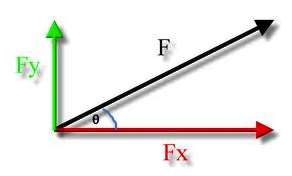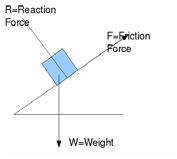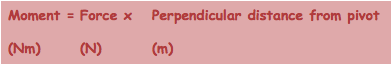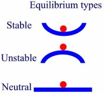# Forces in Equilibrium

Components of forces, moments

Vectors and Scalars

·      A scalar quantity has a magnitude but no direction e.g. mass

·      A vector quantity has a magnitude and direction e.g. velocity

Resultant Vectors

·      This is the single vector that could take the place of two (or more) other vectors. Commonly taken with velocities and forces at AS level

·      Can be found using a scale drawing or mathematically

Scale Drawing

- choose a scale that will fit on your paper

- draw the two (or more vectors) end to end at the correct angles (use a protractor)

- measure the resultant length and convert to a value using your chosen scale

- measure the resultant angle with a protractorMathematically

-for two forces in the same plane

choose a positive direction, in this case, right

The resultant is then the sum in that direction: 200 – 50 = 150N right

-       for two forces at right anglesDraw a simplified diagramComponents of Vectors

·      This is the opposite process to finding the resultant

·      The components are a horizontal and vertical vectors that could be combined to give a resultant of the vector you haveThe horizontal and vertical components of the force F are FX and FY.(draw a triangle with the vectors and use SoCaToa if you need to)

Free Body Diagrams of Forces

·      This is a simplified diagram of an object showing only the forces acting on the object as arrows in the direction the forces act

·      It does not have to be to scale (unless it is to be used to find a resultant force by scale drawing) – however lines are drawn different lengths to denote the magnitude of forces. E.g. longer arrows for larger forces

·      Force arrows are drawn from the point at which they act in the direction of the force

e.g. for an object in equilibria on a slope:Types of Forces

·      Contact forces are those which arise due to the interaction of particles e.g. the support force felt by a person leaning on a desk is provided by the interaction of particles in the persons arms with the particles in the desk (complicated actually – a result of electromagnetic repulsion from the negative electrons in the arms and the table)

Friction is another common contact force

·      Forces due to a field are those which arise because of a property of the object e.g. Some metals are magnetic and so feel a magnetic force – people contain very little of these materials and so do not feel it. Gravitational, Magnetic and Electric fields will be studied at A2 levelWeight is a common example of this kind of force at AS level. Weight is the action of the gravitational field strength of the earth acting on a mass:

Forces in Equilibira

·      Rules arise from Newtons 1st Law of Motion

“Objects remain at rest or in constant uniform motion unless acted upon by an external resultant force”

·      The forces on static objects must therefore be balanced : this means a zero resultant force

·      A scale diagram of the forces forms a closed shape

·It can be usefully broken down into 2 rules for an object in equilibria:Moments

·The moment of a force is its turning effect : this depends not only on the size of the force but also on how far away it is from the pivot point

·      A moment can be in either a clockwise or anti-clockwise direction

·The principle of moments applies to Newtons 1st law again to situations where an object is in equilibria and has balanced moments acting on it:

·      The centre of mass of an object is the point where a single force acting at that point produces no moment.

·A couple is when there are a pair of equal forces acting in opposite directions around a pivot point.Stability and Toppling

·      An object is stable if the line of action of its weight, drawn vertically downward from its centre of mass, passes through its base

·A stable equilibrium is when an object is stable, then displaced from its equilibrium position will return to its original equilibrium position

·      An unstable equilibrium is when it will not return to the equilibrium position